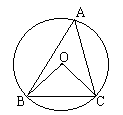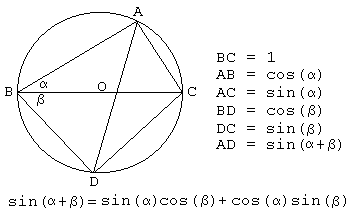# Sine, Cosine, and Ptolemy's Theorem

Ptolemy's theorem implies the theorem of Pythagoras. The latter serves as a foundation of Trigonometry, the branch of mathematics that deals with relationships between the sides and angles of a triangle. In the language of Trigonometry, Pythagorean Theorem reads

$\sin^{2}(A) + \cos^{2}(A) = 1,$

where $A$ is one of the internal angles of a right triangle. If the hypotenuse of the triangle is of length $1,$ then $\sin (A)$ is the length of the side opposite to the angle $A,$ $\cos(A)$ is the length of the adjacent side.

Ptolemy's theorem also provides an elegant way to prove other trigonometric identities. In a little while, I'll prove the addition and subtraction formulas for sine:

(1)

$\sin (A + B) = \sin (A)\cos(B) + \cos(A)\sin (B)$

(2)

$\sin (A - B) = \sin (A)\cos(B) - \cos(A)\sin (B).$

But first let's have a simple proof for the Law of Sines.Proposition III.20 from Euclid's Elements says:

In a circle the angle at the center is double of the angle at the circumference, when angles have the same circumference as base.The more common formulation asserts that an angle circumscribed in a circle is equal to half the central angle that subtends the same chord. (As a corollary, from here it follows that all circumscribed angles subtending the same arc are equal irrespective of their position on the circle. This is Proposition III.21) On the diagram, $\angle BOC = 2\angle BAC (= 2A.)$Drop a perpendicular from $O$ on the side $BC.$ Assuming the radius of the circle is $R,$ $OB = OC = R.$ Also, $\angle BOP = \angle POC.$ In $\Delta BOP,$ $\sin (\angle BOP) = BP/OB = BC/2R.$ Therefore, $BC/\sin (\angle BOP) = 2R.$ When angle $A$ is obtuse, the center $O$ is located outside $\Delta ABC$ and the diagram looks differently. The resulting identity is, however, the same. Repeating these steps with the other two angles $B$ and $C$ of $\Delta ABC$ we get the Law of Sines which in the standard notations appear as

(3)

$\displaystyle\frac{a}{\sin (A)} = \frac{b}{\cos(B)}=\frac{c}{\sin (A)}=2R.$

In the case, where the diameter of the circumscribed circle is 1, we have $a = \sin (A),$ $b = \sin (B),$ and $c = \sin (C).$ This is all we need to apply Ptolemy's theorem. This is of course useful to remember the definition of the sine and cosine functions. In a right triangle, the sine of an acute angle is the ratio of the opposite leg to the hypotenuse; its cosine is the ratio of the adjacent leg to the hypotenuse.Consider a quadrilateral $ABDC$ inscribed into a circle of diameter $1$ so that the diagonal $BC$ serves as a diameter.

From the definition of sine and cosine we determine the sides of the quadrilateral. The Law of Sines supplies the length of the remaining diagonal. The addition formula for sine is just a reformulation of Ptolemy's theorem.To prove the subtraction formula, let the side $BC$ serve as a diameter.As a consequence, we obtain formulas for sine (in one step) and for cosine (in two steps) of complementary angles:

\begin{align} \sin (\frac{\pi}{2} - \alpha ) &= \cos \alpha,\\ \cos(\frac{\pi}{2} - \alpha ) &= \sin \alpha. \end{align}

From these and the addition formulas for sine it is not difficult to derive the addition formulas for cosine:

\begin{align} \cos(\alpha + \beta ) &= \cos(\alpha ) \cos(\beta ) - \sin (\alpha ) \sin (\beta ),\\ \cos(\alpha - \beta ) &= \cos(\alpha ) \cos(\beta ) + \sin (\alpha ) \sin (\beta ). \end{align}

(There are additional simple proofs of those formulas.)

### References

1. E. Maor, Trigonometric Delights, Princeton University Press, 1998### Trigonometry